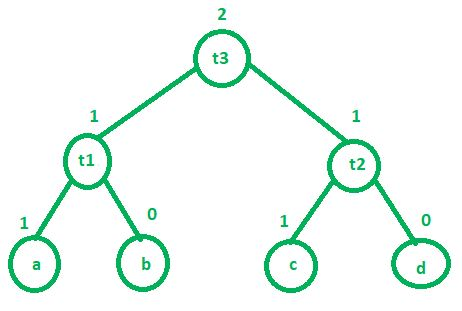# Labeling Algorithm in Compiler Design

Labeling algorithm is used by compiler during code generation phase. Basically, this algorithm is used to find out how many registers will be required by a program to complete its execution. Labeling algorithm works in bottom-up fashion. We will start labeling firstly child nodes and then interior nodes. Rules of labeling algorithm are:

1. If ‘n’ is a leaf node –
• a. If ‘n’ is a left child then its value is 1.
• b. If ‘n’ is a right child then its value is 0.
2. If ‘n’ is an interior node –
Lets assume L1 and L2 are left and right child of interior node respectively.

• a. If L1 == L2 then value of ‘n’ is L1 + 1 or L2 + 1
• b. If L1 != L2 then value of ‘n’ is MAX(L1, L2)

Example:
Consider the following three address code:

```t1 = a + b
t2 = c + d
t3 = t1 + t2 ```Above three address code will require maximum 2 registers to complete its execution.

There is a function called getregister() which is used by compiler to decide where the result will get stored. There are 4 cases of this function which are as follows:

1. If there is a register R which is not holding multiple values then we can use this register to store the value of our result (in the above example, we can store t3 in R, provided that current value in R is not being used anywhere in the program.).
2. If first condition is not satisfied then compiler will search for any empty register to store the value of our result (t3).
3. If there are no empty registers then swap the contents of any registers into the memory and store the result (t3) in that register, provided that those contents do not have any next use.
4. If all the 3 conditions do not hold then store the result in any free memory location.My Personal Notes arrow_drop_upCheck out this Author's contributed articles.

If you like GeeksforGeeks and would like to contribute, you can also write an article using contribute.geeksforgeeks.org or mail your article to contribute@geeksforgeeks.org. See your article appearing on the GeeksforGeeks main page and help other Geeks.

Please Improve this article if you find anything incorrect by clicking on the "Improve Article" button below.

Article Tags :

2

Please write to us at contribute@geeksforgeeks.org to report any issue with the above content.